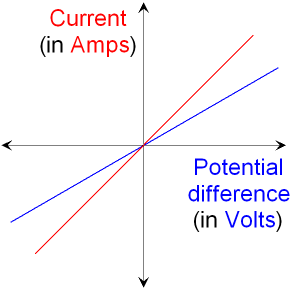gcsescience.com                                       8                                       gcsescience.com

Electricity

What is Resistance?

Resistance is a measure of how much a component
decreases the current (see resistor).
The bigger the resistance, the smaller the current.
Resistance
is measured in ohms (symbol Ω).
See how to calculate the resistance of a component.

What is Ohm's Law?

The very important equation

voltage = current x resistance        V = I x R

is an expression of ohm's law.

If the resistance of a component is constant (constant
means it stays the same) then a plot (graph) of
current against voltage will be a straight line. The gradient
(slope) of the line shows how big the resistance is.

A test circuit is used to find how the current through a
component changes as the voltage changes.
Below is a plot for two components that obey ohm's law.
The component with the blue line shows a smaller
current flowing and therefore has a bigger resistance.Wires and resistors are examples of components that
obey ohm's law. To be precise, a component
will only obey ohm's law at constant temperature.

In reality, an increase in current through
a component will increase its temperature and
so ohm's law is only an approximation
but it works quite well for many components.
See the theory of electrical resistance.

Diodes, lamps and thermistors are
examples of components that do not obey ohm's law.

gcsescience.com        Physics Quiz        Index        Electricity Quiz        gcsescience.com